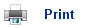# Acids and Bases

• The Bronstead-Lowry theory states that an acid is a proton donor; hydrochloric acid , for example, can donate H+ ions to water forming the oxonium ion:

HCl(aq) + H2O(l)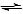H3O+(aq) + Cl-(aq)

• In any acid-base reaction, a conjugate base and conjugate acid is formed: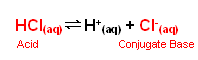• Hydrochloric acid is a strong acid and so the position of equilibrium is far to the right; the HCl is fully dissociated into its ions when added to water; it is a strong acid.
• The strength of an acid refers to the power with which it donates H+ ions to an aqueous solution; strong acids are powerful H+ donors (the donation of H+ ions is almost complete). Conversely, weak acids are less powerful at donating H+ ions, and the reaction with water forming oxonium ions is incomplete.
• The Bronstead-Lowry theory defines a base as a proton acceptor. Ammonia accepts H+ ions when dissolved in water:

NH3(aq) + H2O(l)NH4+(aq) + OH-(aq)

• In this reversible reaction, the position of equilibrium is to the left; ammonia is a weak base.

## Water acting as an acid and a base

• As can be seen from the previous reactions, water can act as both an acid and a base; it is amphoteric.
• The following reaction can occur between water molecules:

H2O(l) + H2O(l)H3O+(aq) + OH-(aq)

Or more simply:

H2O(l)H+(aq) + OH-(aq)

• The position of equilibrium is far to the left in the above reaction; we know that this is the case, as pure water does not conduct electricity; if the position of equilibrium was to the right, then the ions would allow it to conduct electricity.
• As it is an equilibrium reaction, an equilibrium constant can be established: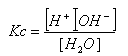• As the position of equilibrium is so far to the left, [H2O] is virtually constant, it can therefore be brought next to the K value: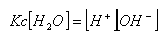• This equilibrium constant is known as the dissociation of water constant, Kw.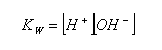• At 298K, this constant has a value of 10-14 mol2 dm-6.
• When an acid or base is added to water, the equilibrium between H+ and OH- is disrupted. The concentrations of OH- or H+ ions in the solution can be calculated using the dissociation constant. For example, in a 0.1 mol dm-3 solution of HCl, the concentration of OH- ions can be calculated: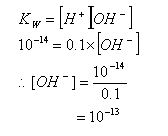• In a 0.02 mol dm-3 solution of NaOH, the concentration of H+ ions can be calculated: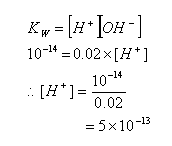• Rather than using very small values, the negative logarithm of the concentration of H+ ions is taken; this gives us the pH of the solution: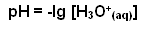• Therefore, the pH of the 0.1 mol dm-3 solution of HCl equates to: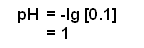• The pH of the 0.02 mol dm-3 solution of NaOH is equal to: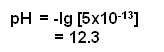• The pH scale allows the strengths of acids and bases to be compared; a strong acid has a low pH; as the pH increases, the solutions become less acidic, then basic and finally become strongly basic.

## Calculating pH values

### Strong acids

• This is a reasonable straight forward calculation; the reaction with water goes to completion, therefore the number of moles of H3O+ ions is equal to the number of moles of acid in the solution.
• Therefore, a 1 mol dm-3 solution of HX (acid) solution will contain 1 mol of H+ ions, therefore its pH will be equal to: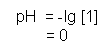• It is important to note the difference with a diprotic acid, such as H2SO4. When added to an aqueous solution, sulphuric acid dissociates as follows:

H2SO4(aq)2H+ (aq) + SO42-(aq)

• Therefore, a 0.01 mol dm-3 solution of H2SO4 will actually dissociate to form 0.02 mols of H+ ions.

### Weak acids

• Weak acids do not fully dissociate in a solution, and so the calculation is more complex.
• Imagine that a HA represents a weak acid; the following equation would illustrates what happens when the acid is added to water:

HA(aq) + H2O(l)H3O+(aq) + A- (aq)

• Once again, an equilibrium constant can be established for this equilibrium reaction: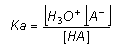• Water can be neglected from the equilibrium constant as it is acting as the solvent.
• The equilibrium constant is called the acidity (dissociation) constant and is given the symbol Ka.
• The table below shows the Ka for some common weak acids: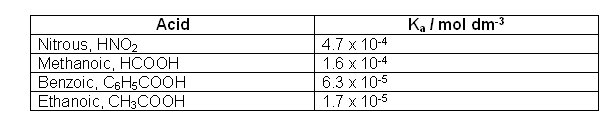• Two assumptions can be made when calculating the [H+]:
1. [H+] = [A-], this may seem obvious as both are formed when HA dissociates; however, water itself is also a source of H+ ions. The amount of H+ ions formed by water is insignificant, thus it is neglected.
2. The amount of HA at equilibrium is equal to the amount of HA put into the solution; in other words, the fraction of HA that has lost an H+ ion can be ignored. This can be done, as we are dealing with weak acids and so this fraction is only very small.
• With these assumptions, it is possible to calculate the pH of a weak acid. For example, the pH of a 0.01 mol dm-3 solution of ethanoic acid can be worked out as follows: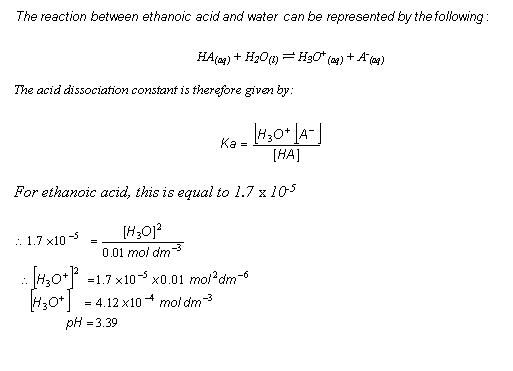## Useful books for revision

Revise A2 Chemistry for Salters (OCR A Level Chemistry B)Salters (OCR) Revise A2 ChemistryHome